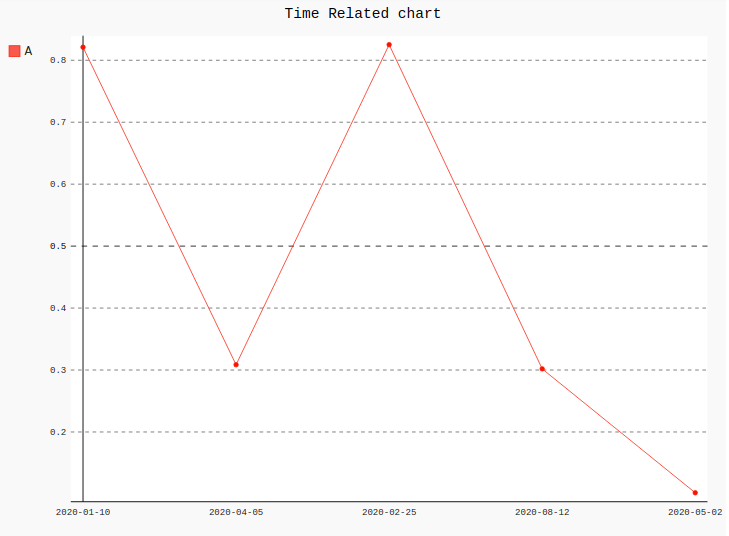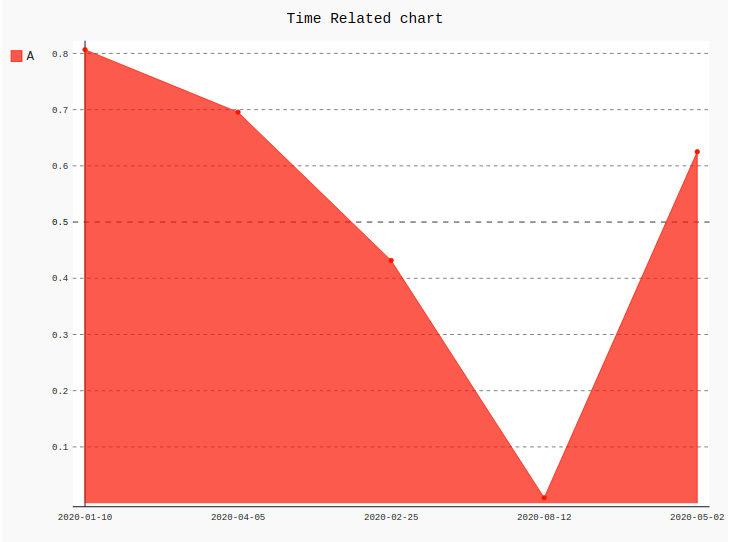# How to Create time related line chart in Pygal?

Pygal is a Python module that is mainly used to build SVG (Scalar Vector Graphics) graphs and charts. SVG is a vector-based graphics in the XML format that can be edited in any editor. Pygal can create graphs with minimal lines of code that can be easy to understand and write.

## Time related chart

Time-related charts can be plotted using the line charts. A line chart or line graph is a type of chart which helps to displays the information as a series of all data points called ‘markers’ and those markers are connected straight through line segments. To plot time-related we have to format the labels. Look at the below examples for better understanding.

Example 1:

 `# importing pygal ` `import` `pygal ` `from` `datetime ``import` `datetime ` `import` `numpy ` ` `  ` `  `# creating line chart object ` `line_chart ``=` `pygal.Line() ` ` `  `# naming the title ` `line_chart.title ``=` `'Time Related chart'` ` `  `# Formatting labels ` `line_chart.x_labels ``=` `map``(``lambda` `d: d.strftime(``'% Y-% m-% d'``), ` `                          ``[datetime(``2020``, ``1``, ``10``), ` `                          ``datetime(``2020``, ``4``, ``5``), ` `                          ``datetime(``2020``, ``2``, ``25``), ` `                          ``datetime(``2020``, ``8``, ``12``), ` `                          ``datetime(``2020``, ``5``, ``2``)]) ` ` `  `# adding lines ` `line_chart.add(``'A'``, numpy.random.rand(``5``)) ` `                           `  `line_chart `

Output:Example 2:

 `# importing pygal ` `import` `pygal ` `from` `datetime ``import` `datetime ` `import` `numpy ` ` `  ` `  `# creating line chart object ` `line_chart ``=` `pygal.StackedLine(fill ``=``"True"``) ` ` `  `# naming the title ` `line_chart.title ``=` `'Time Related chart'` ` `  `# Formatting labels ` `line_chart.x_labels ``=` `map``(``lambda` `d: d.strftime(``'% Y-% m-% d'``), ` `                          ``[datetime(``2020``, ``1``, ``10``), ` `                          ``datetime(``2020``, ``4``, ``5``), ` `                          ``datetime(``2020``, ``2``, ``25``), ` `                          ``datetime(``2020``, ``8``, ``12``), ` `                          ``datetime(``2020``, ``5``, ``2``)]) ` ` `  `# adding lines ` `line_chart.add(``'A'``, numpy.random.rand(``5``)) ` `                           `  `line_chart `

Output:Attention geek! Strengthen your foundations with the Python Programming Foundation Course and learn the basics.

To begin with, your interview preparations Enhance your Data Structures concepts with the Python DS Course.

My Personal Notes arrow_drop_upIf you like GeeksforGeeks and would like to contribute, you can also write an article using contribute.geeksforgeeks.org or mail your article to contribute@geeksforgeeks.org. See your article appearing on the GeeksforGeeks main page and help other Geeks.

Please Improve this article if you find anything incorrect by clicking on the "Improve Article" button below.

Article Tags :

Be the First to upvote.

Please write to us at contribute@geeksforgeeks.org to report any issue with the above content.